Courses

# Fluids and Related Terms Class 11 Notes | EduRev

## JEE : Fluids and Related Terms Class 11 Notes | EduRev

The document Fluids and Related Terms Class 11 Notes | EduRev is a part of the JEE Course Physics For JEE.
All you need of JEE at this link: JEE

FLUID

1. Fluid:

Fluid mechanics deals with the behaviour of fluids at rest and in motion. A fluid is a substance that deforms continuously under the application of a shear (tangential) stress no matter how small the shear stress may be.

Thus, fluids comprise the liquid and gas (or vapor) phase of the physical forms in which matter exists.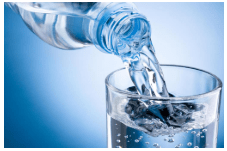Fig: Fluid

Density (r) : Mass of unit volume, Called density

Density at a point of liquid described by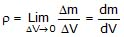density is a positive scalar quantity

SI unit = Kg/m3

CGS unit = gm/cm3

Dimension = [ML-3]

Relative Density: It is the ratio of density of given liquid to the density of pure water at 4°C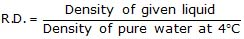Relative density or specific gravity is unit less, dimensionless. It is a positive scalar physical Quantity.

Value of R.D. is same in SI and CGS system due to dimensionless/unitless

Specific Gravity: It is the ratio of weight of given liquid to the weight of pure water at 4°C

Specific Gravity =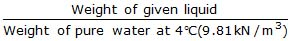=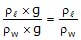= Relative density of liquid i.e. than specfic gravity of a liquid is approximately equal to the relative density. For calculation they can be interchanged.

2. Pressure in a Fluid:

When a fluid (either liquid or gas) is at rest, it exerts a force pependicular to any surface in contact with it, such as a container wall or a body immersed in the fluid.

While the fluid as a whole is at rest, the molecules that makes up the fluid are in motion, the force exerted by the fluid is due to molecules colliding with their surroundings.

If we think of an imaginary surface within the fluid, the fluid on the two sides of the surface exerts equal and opposite forces on the surface, otherwise the surface would accelerate and the fluid would not remain at rest.

Consider a small surface of area dA centered on a point on the fluid, the normal force exerted by the fluid on each side is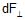. The pressure P is defined at that point as the normal force per unit area, i.e.,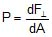If the pressure is the same at all points of a finite plane surface with area A, then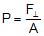where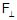is the normal force on one side of the surface. The SI unit of pressure is pascal, where

1 pascal = 1 Pa = 1.0 N/m2

One unit used principally in meterology is the Bar which is equal to 105 Pa.

1 Bar = 105 Pa

2.1 Atmospheric Pressure (P0):

It is pressure of the earth's atmosphere. This changes with weather and elevation. Normal atmospheric pressure at sea level (an average value) is 1.013 × 105 Pa. Thus

1 atm = 1.013 × 105 Pa

Note: Fluid pressure acts perpendicular to any surface in the fluid no matter how that surface is oriented. Hence, pressure has no intrinsic direction of its own, its a scalar. By contrast, force is a vector with a definite direction.

2.2 Variation in Pressure with depth:

If the weight of the fluid can be neglected, the pressure in a fluid is the same throughout its volume. But often the fluid's weight is not negligible and under such condition pressure increases with increasing depth below the surface.

Let us now derive a general relation between the presure P at any point in a fluid at rest and the elevation y of that point. We will assume that the density r and the acceleration due to gravity g are the same throughout the fluid. If the fluid is in equilibrium, every volume element is in equilibrium.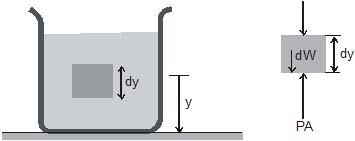Consider a thin element of fluid with height dy. The bottom and top surfaces each have area A, and they are at elevations y and y + dy above some reference level where y = 0. The weight of the fluid element is

dW = (volume) (density) (g) = (A dy) (r) (g)

or dW = rg A dy

What are the other forces in y-direction of this fluid element ? Call the pressure at the bottom surface P, the total y component of upward force is PA. The pressure at the top surface is P dP and the total y-component of downward force on the top surface is (P dP) A. The fluid element is in equilibrium, so the total y-component of force including the weight and the forces at the bottom and top surfaces must be zero.

åFy = 0

PA -(P + dP) A -rgAdy = 0

or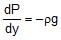...(i)

This equation shows that when y increases, P decreases, i.e., as we move upward in the fluid, pressure decreases.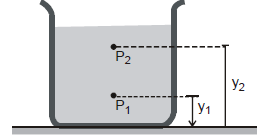If P1 and P2 be the pressures at elevations y1 and y2 and if r and g are constant, then integrating Eq.(i) , we get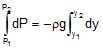or P2 -P1 = -rg (y2 -y1) ...(ii)

It's often convenient to express Eq. (ii) in terms of the depth below the surface of a fluid. Take point 1 at depth h below the surface of fluid and let P represents pressure at this point. Take point 2 at the surface of the fluid, where the pressure is P0 (subscript zero for zero depth). The depth of point 1 below the surface is,

h = y2 -y1

and Eq. (ii) becomes

P0 -P = -rg (y2 -y1) = -rgh

P = P0 rgh ...(iii)

Thus, pressure increases linearly with depth, if r and g are uniform, A graph between P and h is shown below.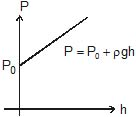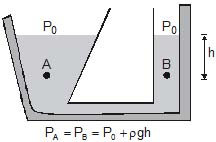Further, the pressure is the same at any two points at the same level in the fluid. The shape of the container does not matter.

2.3. Barometer:

It is a device used to measure atmospheric pressure.

In principle, any liquid can be used to fill the barometer, but mercury is the substance of choice because its great density makes possible an instrument of reasonable size.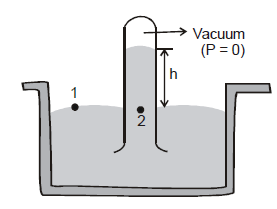P1 = P2
Here,    P1 = atompsheric pressure (P0)
and   P2 = 0 + pgh = p gh
Here,   p = density of mercury
p0 = pgh

Thus, the mercury barometer reads the atmosphereic pressure (P0) directly from the height of the mercury column.

For example if the height of mercury in a barometer is 760 mm, then atmospheric pressure will be,

P0 = rgh = (13.6 × 103) (9.8) (0.760)= 1.01 × 105 N/m2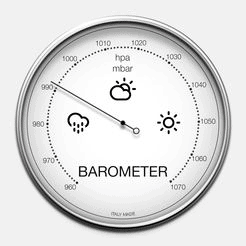Fig: Barometer

2.4 Force on Side Wall of Vessel:

Force on the side wall of the vesel can not be directly determined as at different depths pressures are different. To find this we cosider a strip of width dx at a depth x from the surface of the liquid as shown in figure,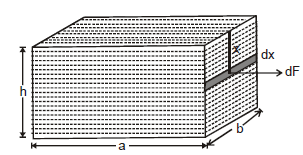and on this strip the force due to the liquid is given as:

dF = xrg × bdx

This force is acting in the direction normal to the side wall.

Net force can be evaluated by integrating equation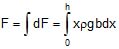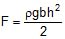...(2.4)

2.5 Average Pressure on Side Wall:

The absolute pressure on the side wall cannot be evaluated because at different depths on this wall pressure is different. The average pressure on the wall can be given as :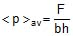=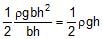...(2.5)

Equation (2.5) shows that the average pressure on side vertical wall is half of the net pressure at the bottom of the vessel.

2.6 Torque on the Side Wall due to Fluid Pressure:

As shown in figure, due to the force dF, the side wall experiences a torque about the bottom edge of the side which is given as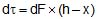= xrgb dx (h -x)

This net torque is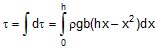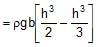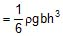2.7 Manometer:

It is a device used to measure the pressure of a gas inside a container.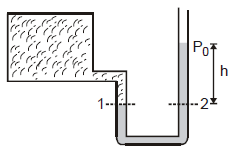The U-shaped tube often contains mercury.

P1 = P2

Here, P1 = pressure of the gas in the container (P)

and P2 = atmospheric pressure (P0) +  rgh

P = P0 hrg

This can also be written as

P -P0 = gauge pressure = hrg

Here, r is the density of the liquid used in U - tube

Thus by measuring h we can find absolute (or gauge) pressure in the vessel.

Ex. 1 Two liquid which do not react chemically are placed in a bent tube as shown in figure. Find out the displacement of the liquid in equilibrium position.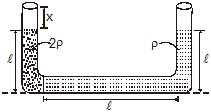Sol. The pressure at the interface must be same, calculated via either tube. Since both tube all open to the atmosphere, we must have.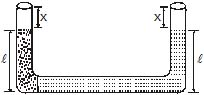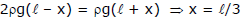Ex. 2 Three liquid which do not react chemically are placed in a bent tube as shown in figure (initially) then fluid out the displacement of the liquid in equilibrium position.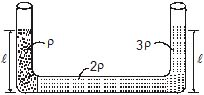Sol. Let us assume that level of liquid having density 3r displaced below by x as shown in figure below.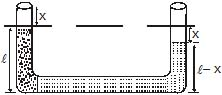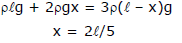2.8 Pressure Distribution in an Accelerated Frame:

We've already discussed that when a liquid is filled in a container, generally its free surface remains horizontal as shown in figure (a) as for its equilibrium its free surface must be normal to gravity i.e. horizontal. Due to the same reason we said that pressure at every point of a liquid layer parallel to its free surface remains constant. Similar situation exist when liquid is in an accelerated frame as shown in figure (b). Due to acceleration of container, liquid filled in it experiences a pseudo force relative to container and due to this the free surface of liquid which normal to the gravity now is filled as: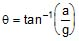...(2.22)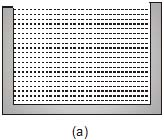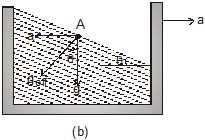Now from equilibrium of liquid we can state that pressure at every point in a liquid layer parallel to the free surface (which is not horizontal), remains same for example if we find pressure at a point A in the accelerated container as shown in figure (a) is given as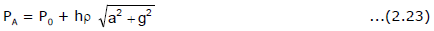Where h is the depth of the point A below the free surface of liquid along effective gravity and P0 is the atmospheric pressure acting on free surface of the liquid.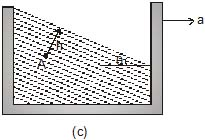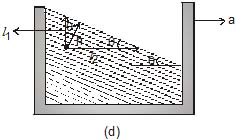The pressure at point A can also obtained in an another way as shown in figure (b). If l1 and l2 are the vertical and horizontal distances of point A from the surface of liquid then pressure at point A can also be given as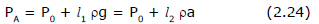Here l1rg is the pressure at A due to the vertical height of liquid above A and according to Pascal's Law pressure at A is given as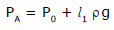...(2.25)

Here we can write l1 as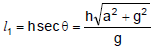or from equation (2.25)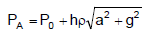Similarly if we consider the horizontal distance of point A from free surface of liquid, which is l2 then due to pseudo acceleration of container the pressure at point A is given as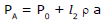...(2.26)

Here l2 is given as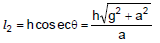From equation (2.24), we have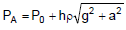Here students should note that while evaluating pressure at point A from vertical direction we haven't mentioned any thing about pseudo acceleration as along vertical length l1, due to pseudo acceleration at every point pressure must be constant similarly in horizontal direction at every point due to gravity pressure remains constant.

Ex. 3 Figure shows a tube in which liquid is filled at the level. It is now rotated at an angular frequency w about an axis passing through arm A find out pressure difference at the liquid interfaces.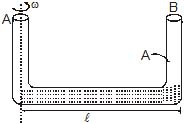Sol. To solve the problem we take a small mass dm from the axis at `a' distance x in displaced condition.

Net inward force = (P +dP) A -PA

= AdP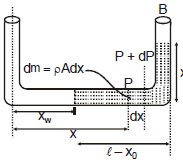This force is balanced by centripetal force in equilibrium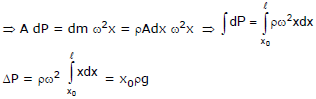Ex. 4 A liquid of density r is in a bucket that spins with angular velocity w as shown in figure.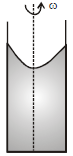Show that the pressure at a radial distance r from the axis is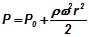where P0 is the atmospheric pressure.

Sol. Consider a fluid particle P of mass m at coordinates

(x, y). From a non-inertial rotating frame of reference two forces are acting on it,

(i) pseudo force (mxw2)
(ii) weight (mg)

in the directions shown in figure.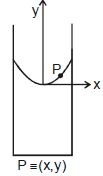Net force on it should be perpendicular to the free surface (in equilibrium). Hence.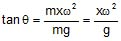or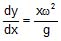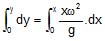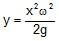This is the equation of the free surface of the liquid, which is a parabola.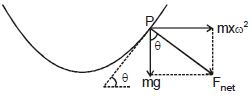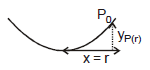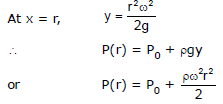Offer running on EduRev: Apply code STAYHOME200 to get INR 200 off on our premium plan EduRev Infinity!

## Physics For JEE

188 videos|491 docs|265 tests

,

,

,

,

,

,

,

,

,

,

,

,

,

,

,

,

,

,

,

,

,

;